# 多分类ROC曲线

### 1 基本概念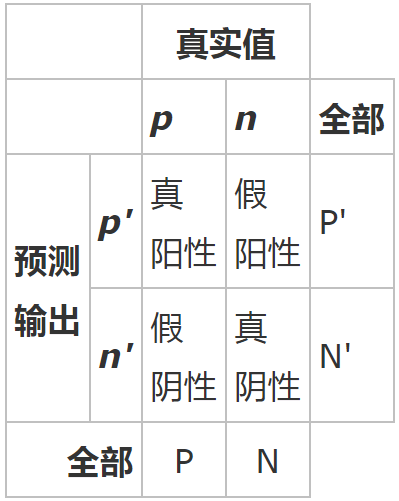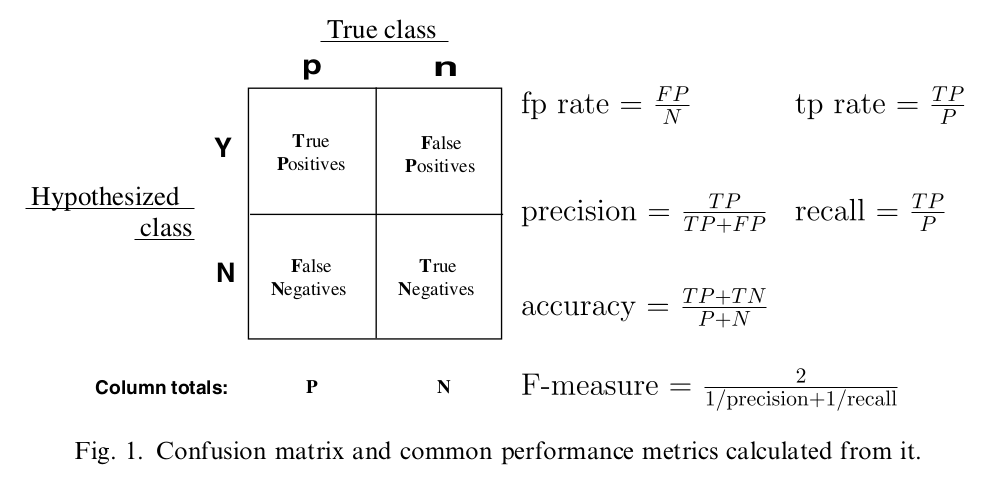precision：预测为对的当中，原本为对的比例（越大越好，1为理想状态）
recall：原本为对的当中，预测为对的比例（越大越好，1为理想状态）
F-measure：F度量是对准确率和召回率做一个权衡（越大越好，1为理想状态，此时precision为1，recall为1）
accuracy：预测对的（包括原本是对预测为对，原本是错的预测为错两种情形）占整个的比例（越大越好，1为理想状态）
fp rate：原本是错的预测为对的比例（越小越好，0为理想状态）
tp rate：原本是对的预测为对的比例（越大越好，1为理想状态）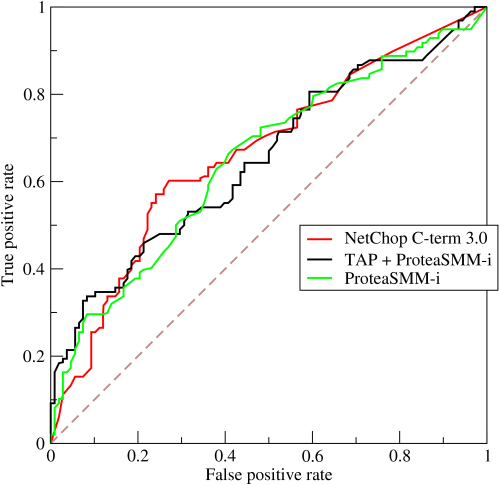### 3 如何画ROC曲线

fpr, tpr, thresholds  =  roc_curve(y_test, scores) 
1

roc_auc =auc(fpr, tpr) 
1

#### （1）二分类问题中的ROC曲线

# -*- coding: utf-8 -*-

import numpy as np
import matplotlib.pyplot as plt
from sklearn import svm, datasets
from sklearn.metrics import roc_curve, auc  ###计算roc和auc
from sklearn import cross_validation

# Import some data to play with
X = iris.data
y = iris.target

##变为2分类
X, y = X[y != 2], y[y != 2]

# Add noisy features to make the problem harder
random_state = np.random.RandomState(0)
n_samples, n_features = X.shape
X = np.c_[X, random_state.randn(n_samples, 200 * n_features)]

# shuffle and split training and test sets
X_train, X_test, y_train, y_test = cross_validation.train_test_split(X, y, test_size=.3,random_state=0)

# Learn to predict each class against the other
svm = svm.SVC(kernel='linear', probability=True,random_state=random_state)

###通过decision_function()计算得到的y_score的值，用在roc_curve()函数中
y_score = svm.fit(X_train, y_train).decision_function(X_test)

# Compute ROC curve and ROC area for each class
fpr,tpr,threshold = roc_curve(y_test, y_score) ###计算真正率和假正率
roc_auc = auc(fpr,tpr) ###计算auc的值

plt.figure()
lw = 2
plt.figure(figsize=(10,10))
plt.plot(fpr, tpr, color='darkorange',
lw=lw, label='ROC curve (area = %0.2f)' % roc_auc) ###假正率为横坐标，真正率为纵坐标做曲线
plt.plot([0, 1], [0, 1], color='navy', lw=lw, linestyle='--')
plt.xlim([0.0, 1.0])
plt.ylim([0.0, 1.05])
plt.xlabel('False Positive Rate')
plt.ylabel('True Positive Rate')
plt.legend(loc="lower right")
plt.show()
1234567891011121314151617181920212223242526272829303132333435363738394041424344454647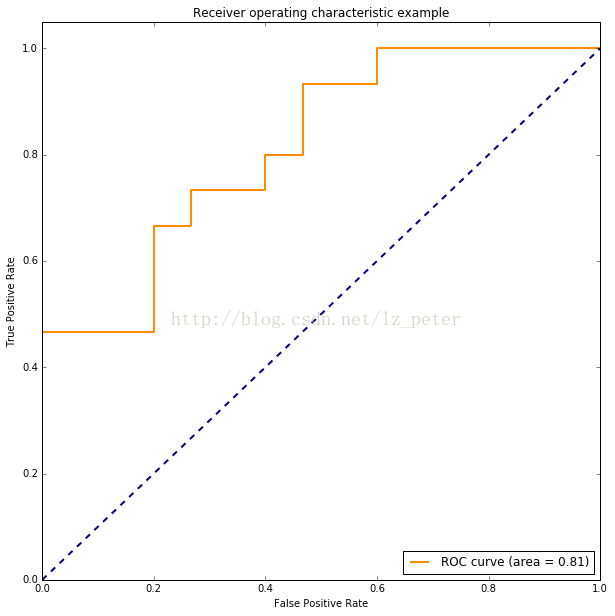#### （2）多分类问题中的ROC曲线

①方法一：每种类别下，都可以得到m个测试样本为该类别的概率（矩阵P中的列）。所以，根据概率矩阵P和标签矩阵L中对应的每一列，可以计算出各个阈值下的假正例率（FPR）和真正例率（TPR），从而绘制出一条ROC曲线。这样总共可以绘制出n条ROC曲线。最后对n条ROC曲线取平均，即可得到最终的ROC曲线。
②方法二：

# 引入必要的库
import numpy as np
import matplotlib.pyplot as plt
from itertools import cycle
from sklearn import svm, datasets
from sklearn.metrics import roc_curve, auc
from sklearn.model_selection import train_test_split
from sklearn.preprocessing import label_binarize
from sklearn.multiclass import OneVsRestClassifier
from scipy import interp

# 加载数据
X = iris.data
y = iris.target
# 将标签二值化
y = label_binarize(y, classes=[0, 1, 2])
# 设置种类
n_classes = y.shape

# 训练模型并预测
random_state = np.random.RandomState(0)
n_samples, n_features = X.shape

# shuffle and split training and test sets
X_train, X_test, y_train, y_test = train_test_split(X, y, test_size=.5,random_state=0)

# Learn to predict each class against the other
classifier = OneVsRestClassifier(svm.SVC(kernel='linear', probability=True,
random_state=random_state))
y_score = classifier.fit(X_train, y_train).decision_function(X_test)

# 计算每一类的ROC
fpr = dict()
tpr = dict()
roc_auc = dict()
for i in range(n_classes):
fpr[i], tpr[i], _ = roc_curve(y_test[:, i], y_score[:, i])
roc_auc[i] = auc(fpr[i], tpr[i])

# Compute micro-average ROC curve and ROC area（方法二）
fpr["micro"], tpr["micro"], _ = roc_curve(y_test.ravel(), y_score.ravel())
roc_auc["micro"] = auc(fpr["micro"], tpr["micro"])

# Compute macro-average ROC curve and ROC area（方法一）
# First aggregate all false positive rates
all_fpr = np.unique(np.concatenate([fpr[i] for i in range(n_classes)]))
# Then interpolate all ROC curves at this points
mean_tpr = np.zeros_like(all_fpr)
for i in range(n_classes):
mean_tpr += interp(all_fpr, fpr[i], tpr[i])
# Finally average it and compute AUC
mean_tpr /= n_classes
fpr["macro"] = all_fpr
tpr["macro"] = mean_tpr
roc_auc["macro"] = auc(fpr["macro"], tpr["macro"])

# Plot all ROC curves
lw=2
plt.figure()
plt.plot(fpr["micro"], tpr["micro"],
label='micro-average ROC curve (area = {0:0.2f})'
''.format(roc_auc["micro"]),
color='deeppink', linestyle=':', linewidth=4)

plt.plot(fpr["macro"], tpr["macro"],
label='macro-average ROC curve (area = {0:0.2f})'
''.format(roc_auc["macro"]),
color='navy', linestyle=':', linewidth=4)

colors = cycle(['aqua', 'darkorange', 'cornflowerblue'])
for i, color in zip(range(n_classes), colors):
plt.plot(fpr[i], tpr[i], color=color, lw=lw,
label='ROC curve of class {0} (area = {1:0.2f})'
''.format(i, roc_auc[i]))

plt.plot([0, 1], [0, 1], 'k--', lw=lw)
plt.xlim([0.0, 1.0])
plt.ylim([0.0, 1.05])
plt.xlabel('False Positive Rate')
plt.ylabel('True Positive Rate')
plt.title('Some extension of Receiver operating characteristic to multi-class')
plt.legend(loc="lower right")
plt.show()
123456789101112131415161718192021222324252627282930313233343536373839404142434445464748495051525354555657585960616263646566676869707172737475767778798081828384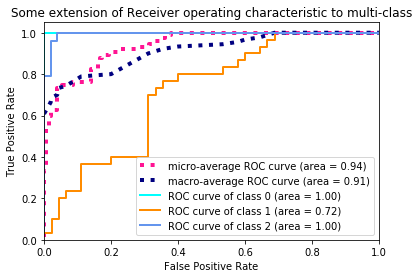plt.figure()
lw = 2
plt.plot(fpr, tpr, color='darkorange',
lw=lw, label='ROC curve (area = %0.2f)' % roc_auc)
plt.plot([0, 1], [0, 1], color='navy', lw=lw, linestyle='--')
plt.xlim([0.0, 1.0])
plt.ylim([0.0, 1.05])
plt.xlabel('False Positive Rate')
plt.ylabel('True Positive Rate')
plt.legend(loc="lower right")
plt.show()
123456789101112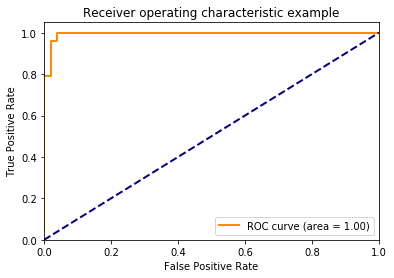### 4 参考文献

【1】(Fawcett, 2006)，Fawcett, T. (2006). An introduction to ROC analysis. Pattern recognition letters, 27(8), 861-874.
【2】Davis, J., & Goadrich, M. (2006, June). The relationship between Precision-Recall and ROC curves. In Proceedings of the 23rd international conference on Machine learning (pp. 233-240). ACM.
【3】ROC和AUC介绍以及如何计算AUC
【4】ROC曲线、AUC、Precision、Recall、F-measure理解及Python实现
【5】ROC曲线
【6】多分类下的ROC曲线和AUC
【7】用Python画ROC曲线03-042万+
10-304278
09-15113
06-144109
10-301609
03-055万+
06-047462
12-22739
08-198万+
09-05865
09-21169
10-17
05-19225
09-17
07-293302
08-31点击重新获取扫码支付余额充值